• 今天小编就为大家分享一篇python实现画出e指数函数图像，具有很好的参考价值，希望对大家有所帮助。一起跟随小编过来看看吧
• ## MATLAB画函数图像

万次阅读 多人点赞 2019-04-19 15:56:34
1 画图基础 （1）一元一次函数 x=0:0.1:1; y=x; plot(x,y); %图像见下图1 图1 ...


1 画图基础
（1）一元一次函数
x=0:0.1:1;
y=x;
plot(x,y);   %图像见下图1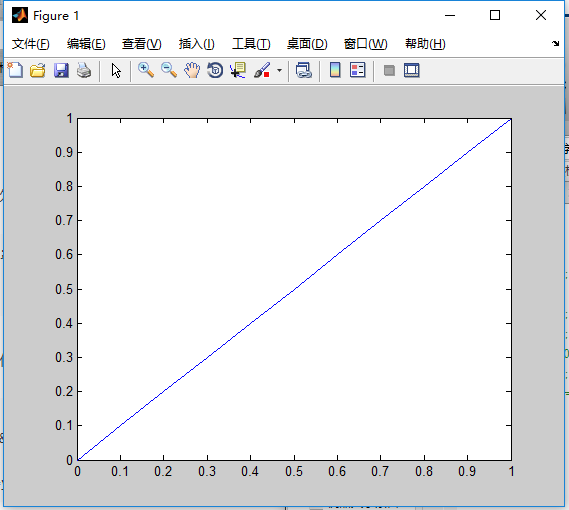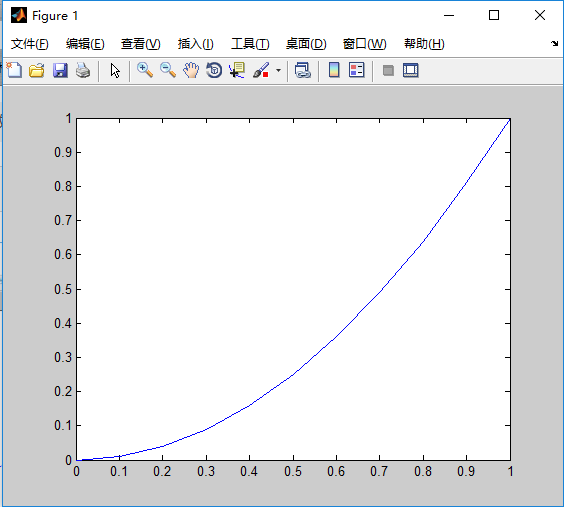图1                                                                                          图2
（2）一元多次函数
 x=0:0.1:1;
y=x.^2;
plot(x,y);    % 图像见图2
2 分段函数图像
x=0:0.1:2;
y=x.*(x>=0&x<=1)+(-(x-1).^2+1).*(x>1&x<=2);
plot(x,y);   %图像见图3
%组合函数y=y1.*(x定义域)+y2.*（x定义域）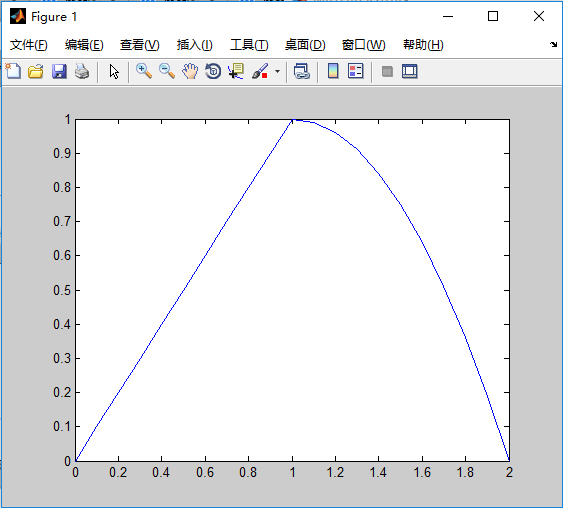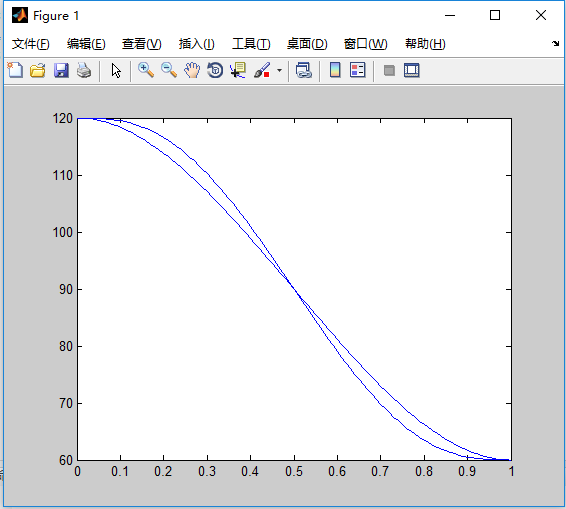图3                                                                                       图4
3 其他小技巧
（1）多条曲线画在同一个图像里
tip：使用 hold on 函数
t=[0:0.01:1];
q1=120-180*t.^2+120*t.^3;
q2=120-600*t.^3+900*t.^4-360*t.^5;
plot(t,q1);hold on;
plot(t,q2);
%图形见图4
（2）改变图像中线的颜色和线条形式（针对plot函数）
t=[0:0.01:1];
q1=120-180*t.^2+120*t.^3;
q2=120-600*t.^3+900*t.^4-360*t.^5;
plot(t,q1,'r');hold on;
plot(t,q2,'b');
%见图5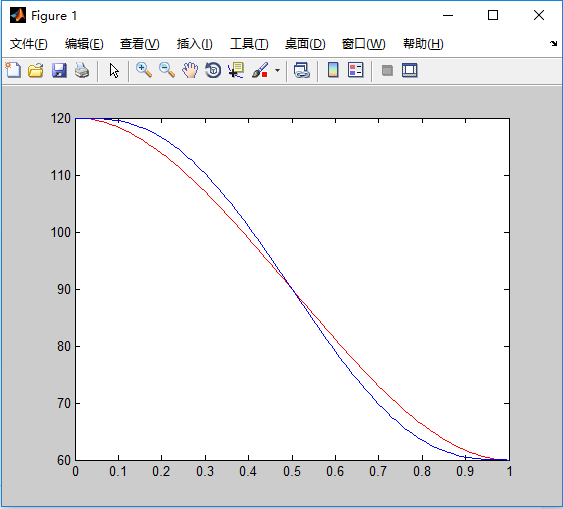图5
通过在plot（）函数括号里面增加特性来改变图线，常见的颜色和类型如图6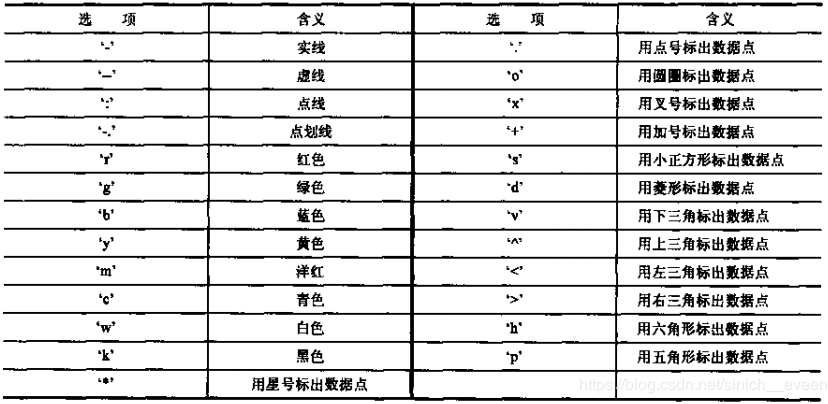图6
（3）增加图例
tip：利用l egend 函数
t=[0:0.01:1];
q1=120-180*t.^2+120*t.^3;
q2=120-600*t.^3+900*t.^4-360*t.^5;
plot(t,q1,'r'); hold on;
plot(t,q2,'b');
legend('a)函数图像','b)函数图像')  %要按函数的顺序来添加
%见图7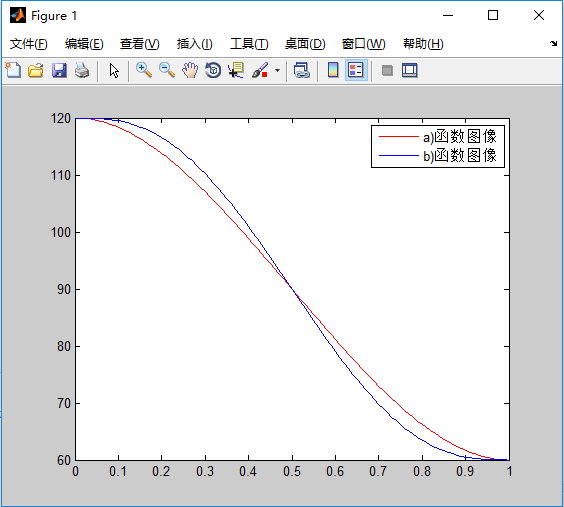图7

展开全文• python语言画出Sigmoid,Tanh,RuLe函数图像,实现图像的可视化
• 易语言画函数图像源码,画函数图像,是否为数字,取两文本中间,转换x,转换y
• 比如 怎么绘制这个函数图像的曲线
比如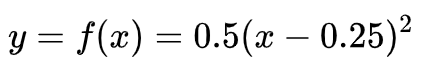怎么绘制这个函数图像的曲线

win10自带的计算器可以解决这个问题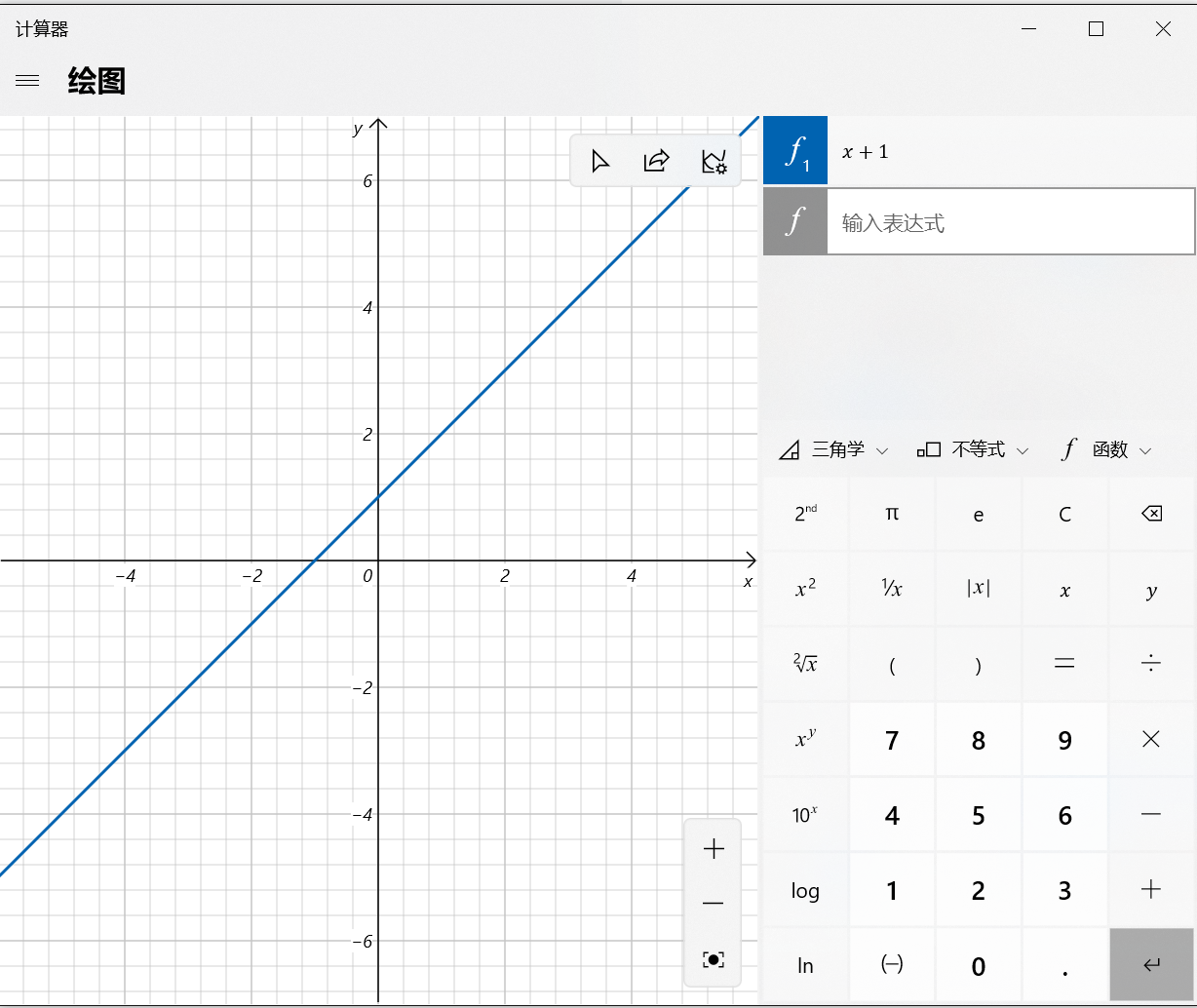展开全文• 基于MFC编写一个单文档程序，在客户区用图形来展现各种数学函数曲线。 具体要求： 1）可绘制常见的数学函数曲线，如三角函数、指数函数、幂函数等。 2）通过菜单选择不同的函数曲线类型，可以通过对话框指定该函数的...mfc
• 1、进入工作目录文件夹D:\Python\jupyterWorkplace 如何截取鼠标右键的选项栏： ...然后选择右键菜单（或者下拉菜单）也可以哦! 这个时候松开shift ,ctrl+alt上的两个...5、画出正弦函数曲线 Ctrl+Enter 运行函数 6、关闭
• * 这是一个简单的函数显示工具，用户输入简单函数，程序自动画出函数图像，有着完整的注释，十分容易理解 * 支持常数e, sin, cos, tan, ln, ^, 加减乘除括号以及它们的复合运算 * 只能有一个变量，而且必须是x，...
• 主要介绍了python 图像处理一个正弦函数代码实例,文中通过示例代码介绍的非常详细，对大家的学习或者工作具有一定的参考学习价值,需要的朋友可以参考下
• python绘制函数图像代码，NumPy(Numerical Python) 是 Python 语言的一个扩展程序库，支持大量的维度数组与矩阵运算，此外也针对数组运算提供大量的数学函数库。 NumPy 的前身 Numeric 最早是由 Jim Hugunin 与其它...python
• 最近因为复习高数在网上找三角函数图像，发现大多数都模糊不清，实在是头痛，所以自己学以致用，用Python画出了三角函数图像。希望我的博客可以帮到大家，也祝考研党们早日上岸，考上理想的学校！ 想了解代码的详细...
• 今天小编就为大家分享一篇使用python绘制二元函数图像的实例，具有很好的参考价值，希望对大家有所帮助。一起跟随小编过来看看吧
• 用matlab二维正态函数图像 坐标网格 [x,y]=meshgrid(-5:0.1:5,-5:0.1:5) 正态分布密度函数 f=1/(2*pi*sigma1*sigma2*sqrt(1-p*p))*exp(-1/(2*(1-p*p))*(((x-u1).^2)./(sigma1*sigma1)-2*p*((x-u1)*(y-u2))./...正态分布
• 在学校太闲，就写了这个程序，可以绘制函数图像，并且可以绘制其导函数图像和不定积分的图像，效果非常不错。 效果图 说明 1，程序无法绘制复数图像，若函数返回一个复数，将自动取模作为函数值进行绘制。 2，函数...
• 这里用Python逼近函数y = exp(x);同样使用泰勒函数去逼近:exp(x) = 1 + x + (x)^2/(2!) + .. + (x)^n/(n!) + ...#!/usr/bin/python# -*- coding:utf-8 -*-import numpy as npimport mathimport matplotlib as mpl...
这里用Python逼近函数y = exp(x);同样使用泰勒函数去逼近:
exp(x) = 1 + x + (x)^2/(2!) + .. + (x)^n/(n!) + ...
#!/usr/bin/python
# -*- coding:utf-8 -*-
import numpy as np
import math
import matplotlib as mpl
import matplotlib.pyplot as plt
def calc_e_small(x):
n = 10
f = np.arange(1, n+1).cumprod()
b = np.array([x]*n).cumprod()
return np.sum(b / f) + 1
def calc_e(x):
reverse = False
if x < 0: # 处理负数
x = -x
reverse = True
ln2 = 0.69314718055994530941723212145818
c = x / ln2
a = int(c+0.5)
b = x - a*ln2
y = (2 ** a) * calc_e_small(b)
if reverse:
return 1/y
return y
if __name__ == "__main__":
t1 = np.linspace(-2, 0, 10, endpoint=False)
t2 = np.linspace(0, 3, 20)
t = np.concatenate((t1, t2))
print(t) # 横轴数据
y = np.empty_like(t)
for i, x in enumerate(t):
y[i] = calc_e(x)
print('e^', x, ' = ', y[i], '(近似值) ', math.exp(x), '(真实值)')
# print '误差：', y[i] - math.exp(x)
plt.figure(facecolor='w')
mpl.rcParams['font.sans-serif'] = [u'SimHei']
mpl.rcParams['axes.unicode_minus'] = False
plt.plot(t, y, 'r-', t, y, 'go', linewidth=2)
plt.title(u'Taylor展式的应用 - 指数函数', fontsize=18)
plt.xlabel('X', fontsize=15)
plt.ylabel('exp(X)', fontsize=15)
plt.grid(True)
plt.show()
以上这篇python实现画出e指数函数的图像就是小编分享给大家的全部内容了，希望能给大家一个参考，也希望大家多多支持。
展开全文• Java刚开始学习，根据JFrame和JPanel以及Math类编写的小程序，抛砖引玉
• 目的: 发出 y = x ** 3 / (x * 3 + 1) 的图像 1. 导入matplotlib模块还有numpy模块: import numpy as np import matplotlib.pyplot as plt 2. di'y
目的: 发出 y = x ** 3 / (x * 3 + 1) 的图像
1. 导入matplotlib模块还有numpy模块:
import numpy as np
import matplotlib.pyplot as plt
2. 设置一个x的大致范围, 和y相对应的值域:
x = np.arange(-100, 100, 0.001)
y = x ** 3 / (3 * x + 1)

3. 使用figure创建画布, 把参数传进plot里面:
plt.figure()
plt.plot(x, y, linestyle='--', color='red')
4. 因为这个函数的范围太大, 并且在三分之一处出现断崖分布, 必须得缩小x轴和y轴的范围, 不然画图的误差会相当大:
lt.xlim((-0.6, 0.6))
plt.ylim((-0.6, 0.6))
5. 对图像进行些许的美化:
ax = plt.gca()
ax.spines['right'].set_color('none')
ax.spines['top'].set_color('none')
ax.xaxis.set_ticks_position('bottom')
ax.yaxis.set_ticks_position('left')
ax.spines['bottom'].set_position(('data', 0))
ax.spines['left'].set_position(('data', 0))
6. 最后要使用show展示出来:
plt.show()
7. 效果如下: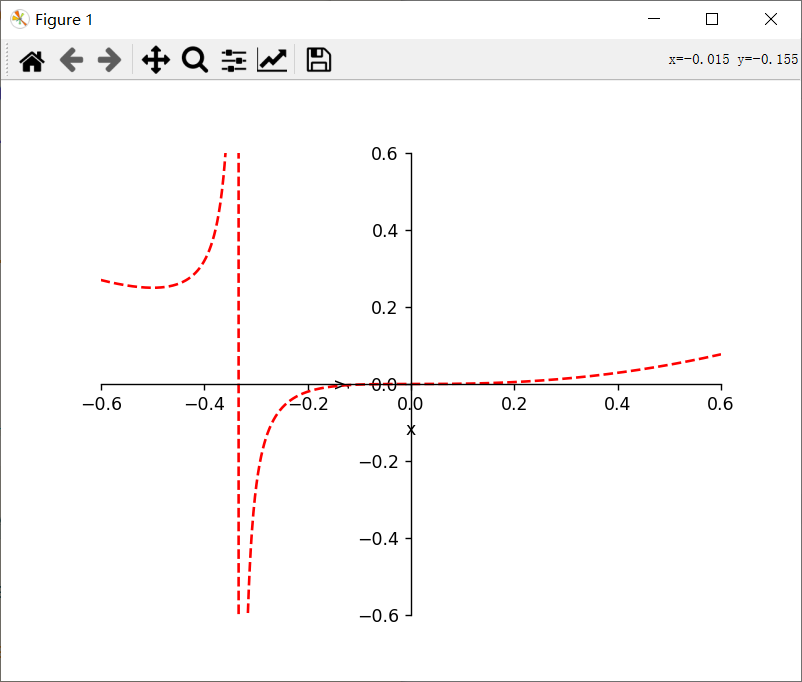8. 所用代码:
import numpy as np
import matplotlib.pyplot as plt

x = np.arange(-100, 100, 0.001)
y = x ** 3 / (3 * x + 1)

plt.figure()
plt.plot(x, y, linestyle='--', color='red')
plt.xlim((-0.6, 0.6))
plt.ylim((-0.6, 0.6))
plt.xlabel('x')
plt.ylabel('y')
ax = plt.gca()
ax.spines['right'].set_color('none')
ax.spines['top'].set_color('none')
ax.xaxis.set_ticks_position('bottom')
ax.yaxis.set_ticks_position('left')
ax.spines['bottom'].set_position(('data', 0))
ax.spines['left'].set_position(('data', 0))
plt.show()


展开全文python
• mesh函数的定义及应用，文件包括了函数的调用，matlab变量的定义
• python 画出函数图像 准备工作 搭建一个jupyter notebook, 浏览器版本的，这样就可以在任意地方继续你的工作 Linux 云服务器，安装 python3 ，jupyter，anaconda 等等 初步概念 Matplotlib 是 Python 的绘图库 ...python 数据分析
• Python如何画函数的曲线输入以下代码导入小编们用到的函数库。 >>> import numpy as np >>> import matplotlib.pyplot as plt >>> x=np.arange(0,5,0.1); >>> y=np.sin(x); plt.plot(x,y) 采用刚才代码后有可能无法...
• 然后把给定函数f(x)在指定区间里的点 (x, f(x)) 依次画出，步进间隔小到一定程度就会连成f(x)对应的曲线。 for ... in range()的步进是整数，所以引入numpy库的arange()，其步进可以是小数，例： for i in numpy.a...python tkinter Canvas 参数方程
• ## python画函数图像

千次阅读 2019-02-05 14:16:03
import numpy as np import matplotlib.pyplot as plt import warnings warnings.filterwarnings('...#指数函数 #f(x) = a**x x = np.linspace(-2,2,100) y1 = (1/2)**x y2 = (1/4)**x plt.scatter(0,1,marker='...
• 主要介绍了Python绘制正余弦函数图像的方法，小编觉得挺不错的，现在分享给大家，也给大家做个参考。一起跟随小编过来看看吧
• sigmoid激活函数： import numpy as np import matplotlib.pylab as plt def sigmoid(x): return 1/(1+np.exp(-x)) # sigmoid函数 x = np.arange(-6.0,6.0,0.1) # 限定x的范围，给什么区间出来的就是在哪个...python 开发语言 后端
• cw模糊度函数，可以画出cwcw脉冲函数模糊度图像...